## Two particles, one with charge − 7.97 μC and one with charge 3.55 μC, are 6.59 cm apart. What is the magnitude of the force that one particl

Question

Two particles, one with charge − 7.97 μC and one with charge 3.55 μC, are 6.59 cm apart. What is the magnitude of the force that one particle exerts on the other?

in progress 0
2 weeks 2021-09-02T20:17:57+00:00 1 Answers 0 views 0

58.6 N

Explanation:

We are given that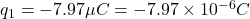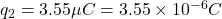Using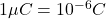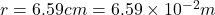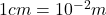The magnitude of force that one particle exerts on the other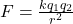Where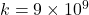Substitute the values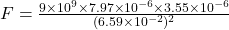F=58.6 N